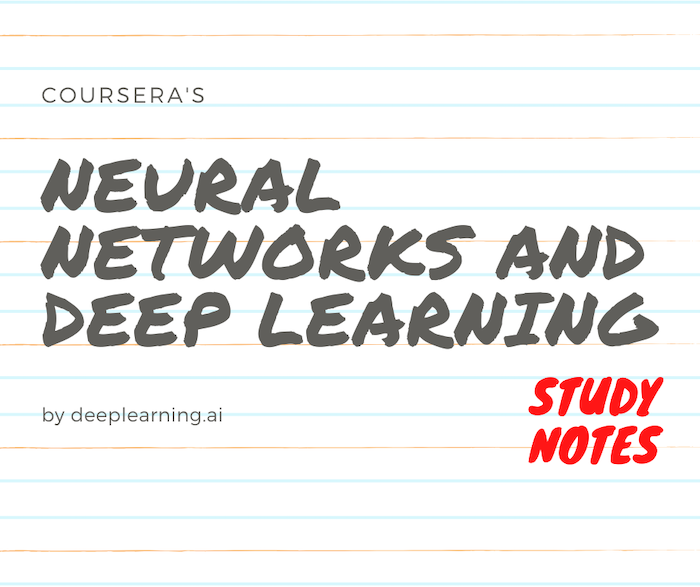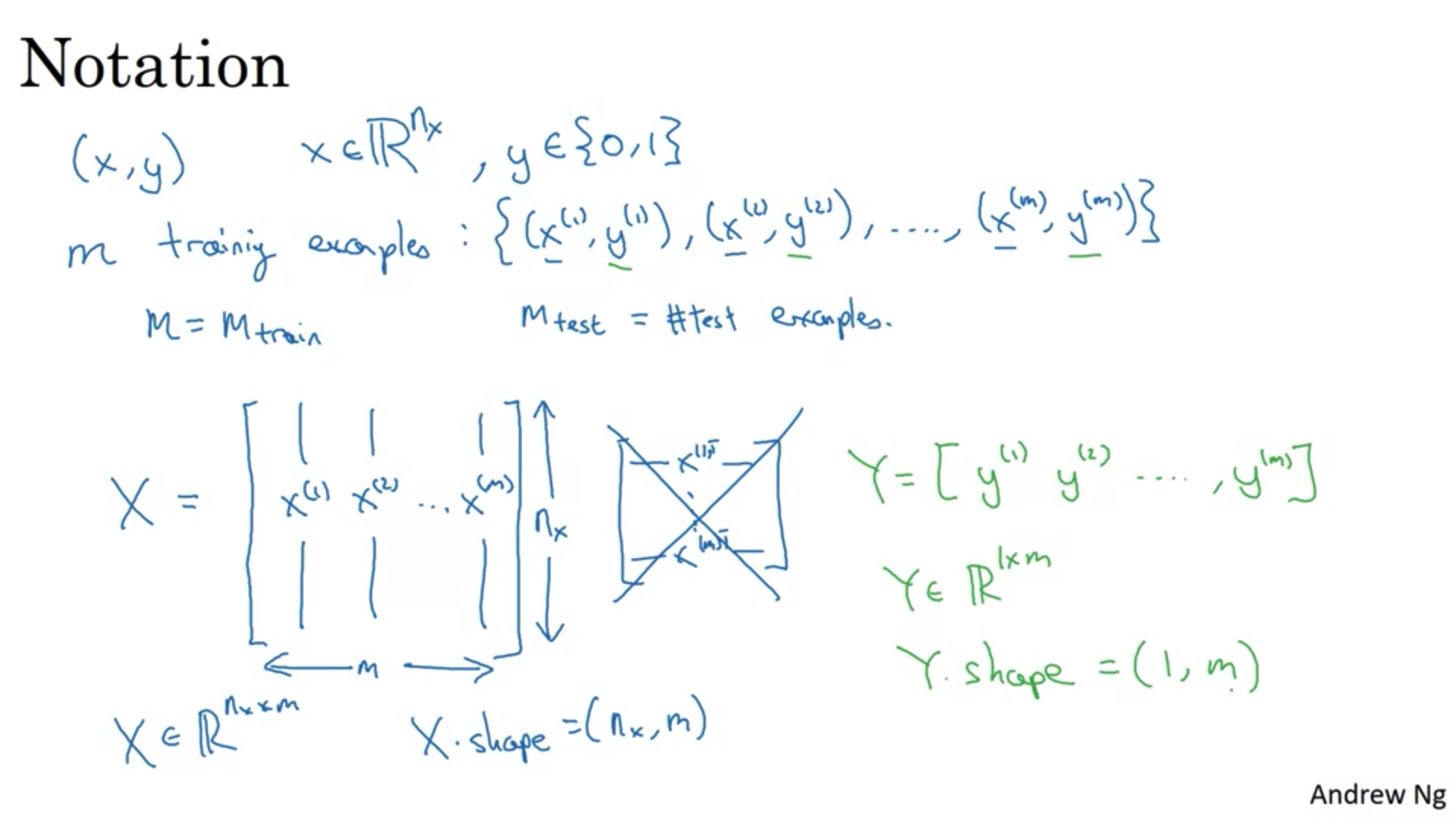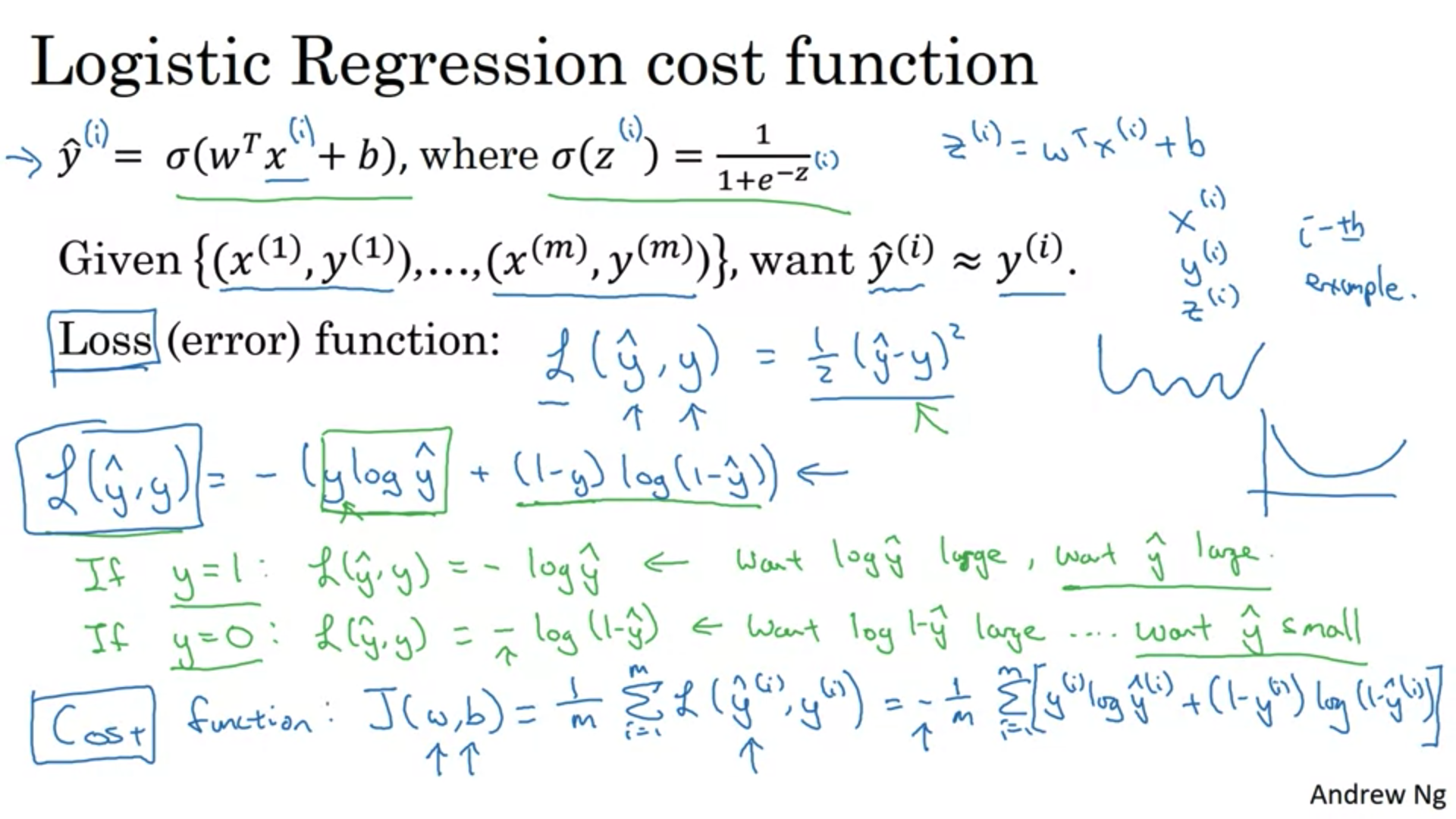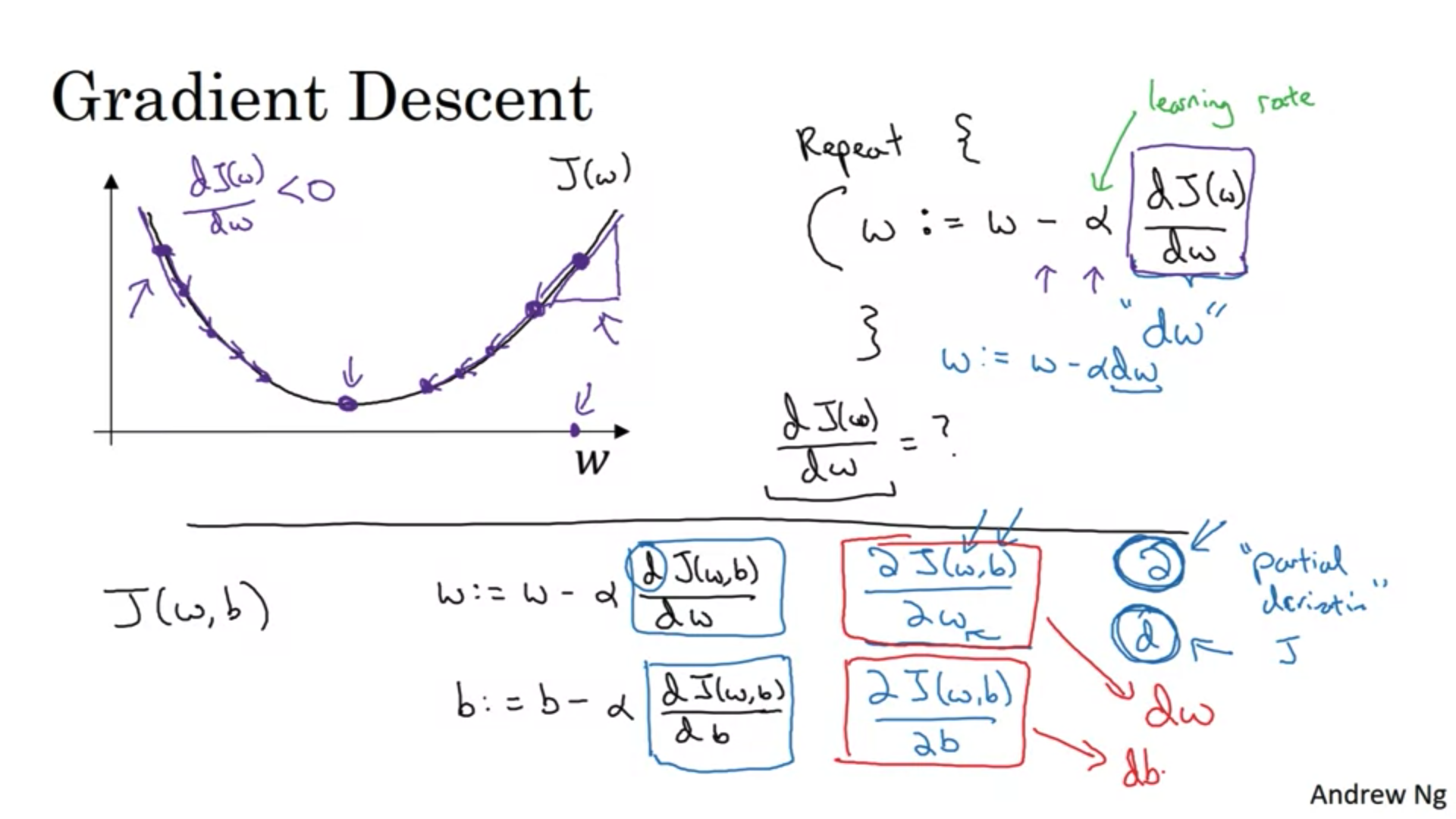# Deeplearning.ai Neural Networks and Deep Learning Week 1 & Week 2# Week 2

## Logistic Regression as a Nerual Network

Logistic regression is an algorithim for binary classification. A binary classification problem that a neural network could solve is a cat calssifier that tells from an image wether there is a cat present or not, to handle images for binary classification, it should be converted into feature vectors.

i.e) an image of 64px is converted into a feature vector of 64X64X3 size = 12288 (n), since its RGB values are going to be matrices of 64X64 size.

### Notation• Training example -> (x,y), where x belongs to the feature vector of size n and y belongs to the output vector of values of either {0,1}.

• m -> training examples set : {(x^(1),y^(1)),(x^(2),y^(2)…..)}

• X -> feature matrix, where each column is a single training example’s feature vector values X^(1), X^(2), X^(3), and each row is a single feature’s values across the different training examples. so the feature matrix size = m (# of training examples) x nx (number of features in each feature vector)

• Y -> output matrix, is a matrix of size 1xm, it’s basically a matrix of one row and multiple columns that constitutes the outputs per training example y^(1),y^(2),y^(3)…y^(m)

### Logistic RegressionIn logistic regression we take an input which is x that belongs to a feature vector of size nx and we want to output a value either 0 or 1, this can be an indication of the probability of the occurance of a certain event (a photo that has a cat in it), given a certain x as an input y^ = P(y=1|x) and with having w and b as parameters to these inputs that can affect this probability or y^ ,the output must be dependant on w and b. but multiplying the transpose of w with X and adding b vector will yield numbers that can be bigger than 1 or smaller than 0, so to limit the output to these two duels we insert our equation into a sigmoid function that evaluates the output into either a 0 or a 1 depending on w and b in our equation which now we call it Z as an input to the sigmoid function.

### Cost Functionwhile training our algorithm to compute the best w and b parameter values, we need to make it adjust to the parameters that give the least error. by denoting y^ as the output computed by the algorithm and y as the true output in our data set, we define out loss function to be the metric of error, and to minimize it, the algorithm adjusts the parameters (w & b) to yield the y^ that’s close enough to y as possible : L(y^,y) = - (ylog y^ + (1-y) log (1-y^)).

but this will calculate the error per training example, if we want to compute the error for the whole training set, we would then define a cost function, a cost function value is big, when the error per training example increase, so it penalize the algorithm in order for it to adjust to a smaller error and thus a smaller cost function.

it’s defined as the summation of the errors for each training example averaged over their number and it gives an indication about how fit are our parameters w and b for computing outputs that are as close to the true outputs as possible.Is basically changing the value of the parameters that gives us the least error (cost function), we randomly initialize our parameters at first and then move in the direction (values of w and b ) that gives us the least error.
w:= w - (alpha) dJ(w)/dw, where alpha is the learning rate that determines the magnitude of these strides we take to minimize the error (controls how fast the values change)
the intution behind this is that for a given w value, if at a given value it yields a high result for J, then the derivative of J with respect to this w value is gonna be positive (positive slope) so subtracting it from w will decrease the value of w and b used untill we reach a value at which J(w,b) is at its minimum.